# How to use the CORREL function in Google SheetsWhen you’re carrying out data analysis, statistical measures help you to understand underlying trends. One of the most common statistical metrics is correlation. It’s used to gauge the extent of the relationship, or dependence, of two variables.

The measure of correlation is called the correlation coefficient. If you have two sets of variable data, you can calculate the Pearson product-moment correlation coefficient (r) using the CORREL function in Google Sheets.

### Measuring correlation in Google Sheets

The Pearson product-moment correlation coefficient (also referred to as Pearson’s r, or simply r) measures the strength of the linear association between two variables.

The correlation coefficient r has a value of between −1 and 1.

• When r = 1, it indicates that the two variables are in a perfect linear relationship. In this case, if x increases, y will increase by the same amount.
• When r = 0, it suggests that there is no correlation between the variables.
• A value of −1 implies that the variables are in a perfectly correlated negative relationship. This means that if x increases, the value of y decreases by a proportionate amount, and vice versa.

The three graphs below will help you get a better understanding of correlation.

#### Positive correlation

This positively correlated data has a correlation coefficient of about 0.9 – close to the maximum of 1. If the correlation were truly 1, then the data would be in a perfectly straight line.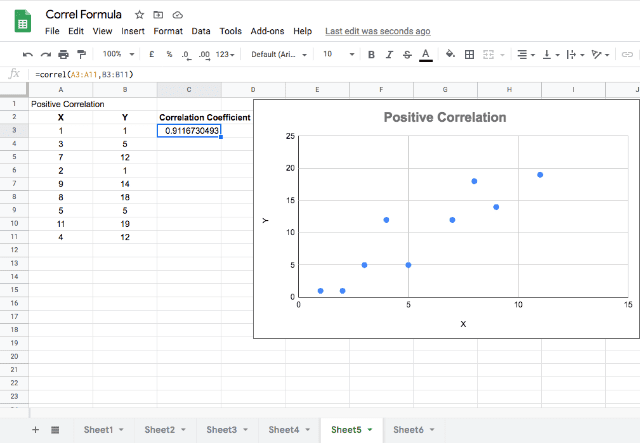#### Negative correlation

The negatively correlated data has a coefficient of about -0.9, which means that the data is well-correlated, but in a negative relationship. So in this case, y decreases as x increases.

In both the positive and negative correlations, it’s possible to draw a “line of best fit” through the data points. This line would give a fairly accurate representation of the data.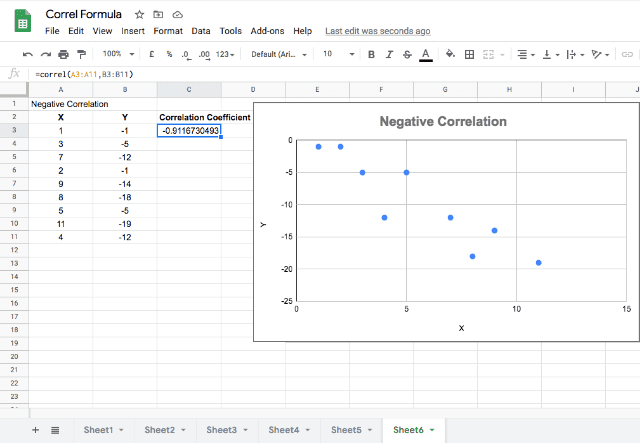#### No correlation

In the graph below, you can see non-correlated data with a correlation coefficient of about 0.04. This indicates that there is no meaningful relationship between the x and y variables.

You would have a hard time drawing any sort of line of best fit through these data points!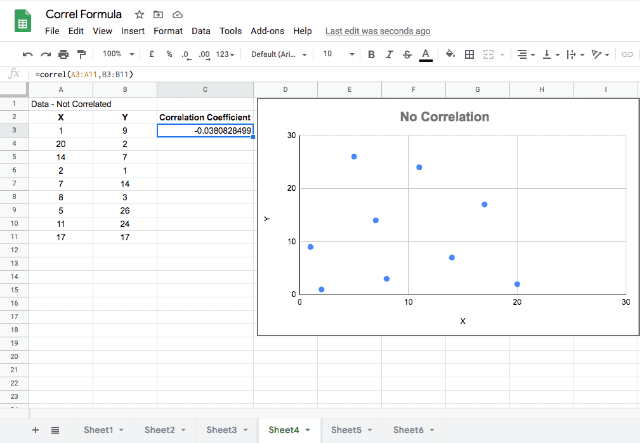If you want to read more about the Pearson product-moment correlation coefficient and how to calculate it, here’s the link.

### Syntax

=CORREL(data_y, data_x)

• data_y – is the range of values or a reference to the range of cells that contain the dependent data.
• data_x – is the range of values or a reference to the range of cells that contain the independent data.

As you can see, there are two options for inputting the dataset. You can use a reference range, or input the data directly into the function.

Generally, using a reference range is a better option. That’s because your data is probably already in the spreadsheet. Using a reference range means you won’t have to re-type it in the function itself.

If you do choose to type the dataset directly into the formula, make sure you enclose both the x and y data in curly brackets {}. If you’re referencing the data in a range of cells, you don’t need to use curly brackets.

### How to use the correlation function in Google Sheets

In the syntax, you can see that you need two sets of data (x and y) for the CORREL function to return a value.

I’ll now show you some practical examples in Google Sheets.

#### Correlation using brackets

The parameters for the CORREL function accept the input values in two different ways.

You can choose to input the direct numeric datasets using curly brackets that indicate ranges.

As you can see, this is what I’ve done in the example in row 2.  Here I have typed in the formula =Correl({8.4,8,1.8,7.2,0.9},{14,20,6,12,3}).

#### Correlation using range references

Alternatively, you can simply use range references. You can see that in the example in row 3, where I’ve typed =Correl(A2:A11,B2:B11).

In both examples, you get a correlation coefficient that is positive and close to 1. While interpretations vary depending on the application and the nature of the data, the first correlation coefficient of 0.91 indicates that the data is closely correlated. So, when x changes, y changes in a very similar way.

The second correlation coefficient of 0.75 indicates that the data is less correlated than the first dataset — but still fairly correlated.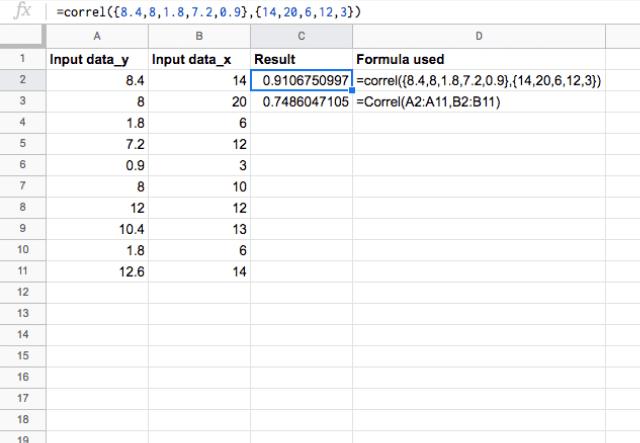#### Positive relationship

Now, you’ll see how the function behaves if the x and y data are in a linear relationship (y = 10x + 5).

In the following image, I’ve added data that fits this relationship. Since this is a direct dependency, the function returns 1 for the correlation coefficient.

Remember, a value of 1 indicates that the data is perfectly correlated.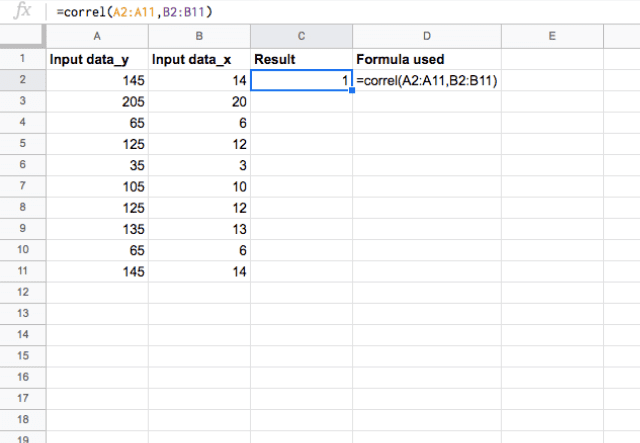#### Negative relationship

What happens when I try an equation with a negative slope (y = -5x+10)?

In this case, the correlation coefficient should be -1, because this is a negative dependency. So when x increases, y decreases by a proportional amount.

You can see in the snapshot below that this is indeed the case.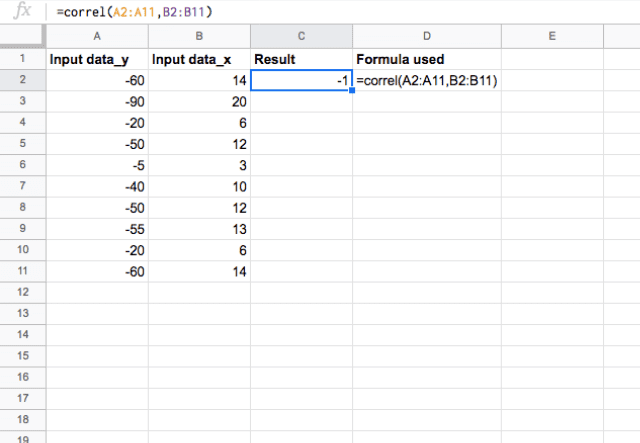That’s the Sheetgo guide on how to calculate a correlation in Google Sheets — thanks for reading! Looking for more advanced Google Sheets tips? Check out our other posts such as how to import Google Sheets data from one sheet to another or how to use QUERY with Importrange.

#### Did you like this post?

Share it with your colleagues, friends, and fellow spreadsheet users via the social media buttons on the left.

Editor’s note: This is a revised version of a previous post that has been updated for accuracy and comprehensiveness.